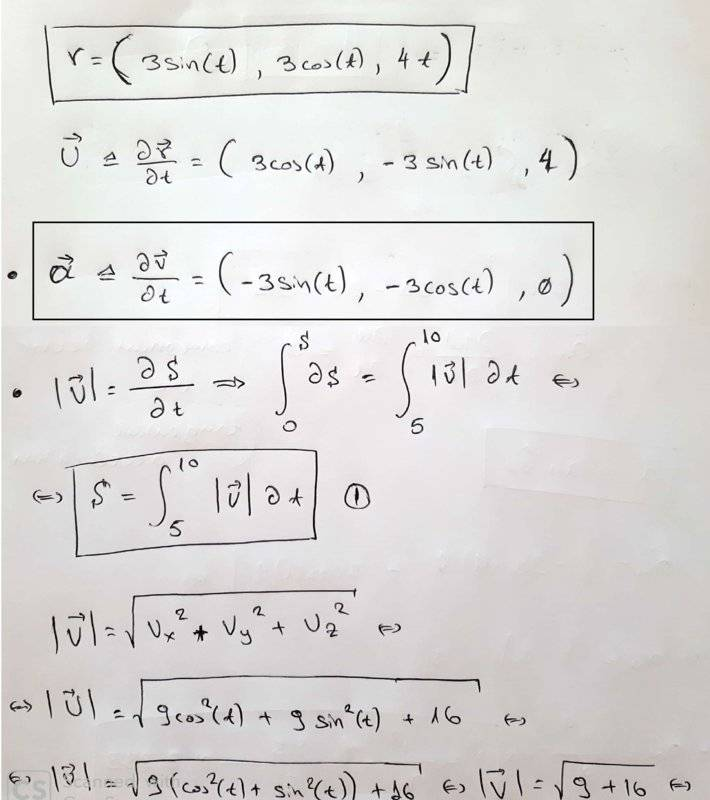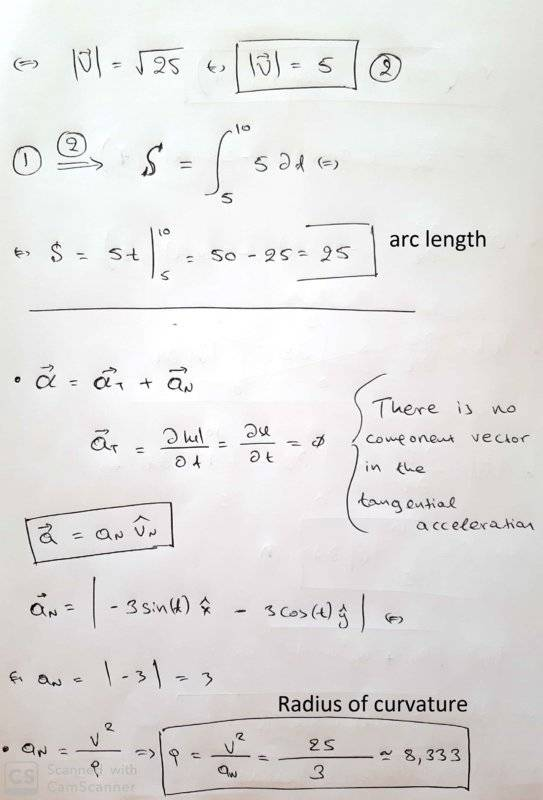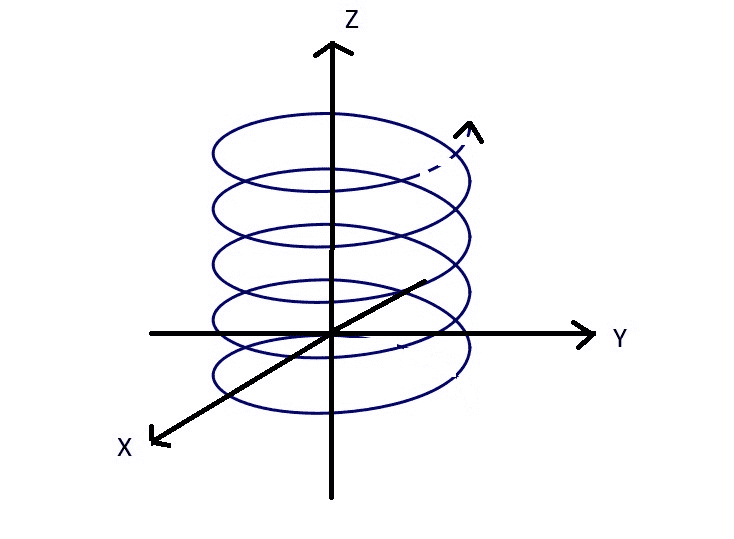# Helicoidal movement: acceleration vector, arc length, radius of curvature

Homework Statement:
The position of a material point in a Cartesian coordinate system 0XYZ given from the equation r=( 3sin(t) , 3cos(t) , 4t ), t : time. Describe in a few words the motion and find the route's length (arc length) from t=5 to t=10. Calculate the accelerattion vector and the radius of curvature at every point on the track.
Relevant Equations:
r = ( 3sin(t) , 3cos(t) , 4t )
I have tried to solve it and I would like a confirmation, correction or if something else is suggested... :)Helicoidal movement

•PeroK

•